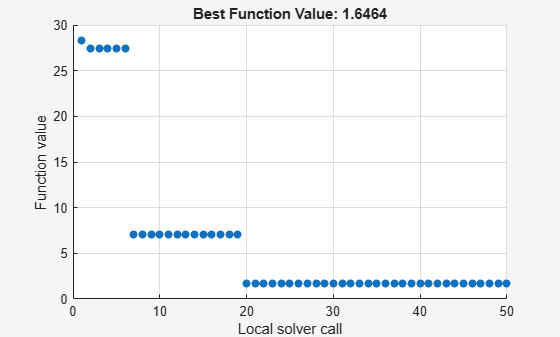# `MultiStart` with `lsqnonlin`, Problem-Based

This example shows how to fit a function to data using `lsqnonlin` together with `MultiStart` in the problem-based approach.

Many fitting problems have multiple local solutions. `MultiStart` can help find the global solution, meaning the best fit.

The model is

$y=a+b{x}_{1}\mathrm{sin}\left(c{x}_{2}+d\right)$,

where the input data is $x=\left({x}_{1},{x}_{2}\right)$, and the parameters a, b, c, and d are the unknown model coefficients.

### Create Problem Data

Most problems involve data from measurements. For this problem, create artificial data including noise. Create 200 data points and responses. Specify the values $a=-3$, $b=1/4$, $c=1/2$, and $d=1$.

```rng default % For reproducibility fitfcn = @(p,xdata)p(1) + p(2)*xdata(:,1).*sin(p(3)*xdata(:,2)+p(4)); N = 200; % Number of data points preal = [-3,1/4,1/2,1]; % Real coefficients xdata = 5*rand(N,2); % Data points ydata = fitfcn(preal,xdata) + 0.1*randn(N,1); % Response data with noise```

### Create Optimization Variables and Problem

The optimization variables are the model coefficients. Set bounds for the variables as you create them. The variable $d$ does not need to exceed $\pi$ in absolute value, because the sine function takes values in its full range over any interval of width $2\pi$. Assume that the coefficient $c$ must be smaller than 20 in absolute value, because allowing a high frequency can cause unstable responses or inaccurate convergence.

```a = optimvar("a"); b = optimvar("b"); c = optimvar("c",LowerBound=-20,UpperBound=20); d = optimvar("d",LowerBound=-pi,UpperBound=pi); prob = optimproblem;```

### Create Objective Function

Create the objective function as the sum of squared differences between the model response and the data.

```resp = a + b*xdata(:,1).*sin(c*xdata(:,2) + d); prob.Objective = sum((resp - ydata).^2);```

### Create Initial Point and Solve Problem

The initial point is a structure with values for the coefficients. Arbitrarily set the initial point to [5,5,5,0].

```x0.a = 5; x0.b = 5; x0.c = 5; x0.d = 0; [sol,fval] = solve(prob,x0)```
```Solving problem using lsqnonlin. Local minimum possible. lsqnonlin stopped because the final change in the sum of squares relative to its initial value is less than the value of the function tolerance. ```
```sol = struct with fields: a: -2.6149 b: -0.0238 c: 6.0191 d: -1.6998 ```
```fval = 28.2524 ```

`lsqnonlin` finds a local solution that is not particularly close to the model parameter values (–3,1/4,1/2,1).

### Search for Improved Solution Using `MultiStart`

To search for a better solution, create a `MultiStart` object. Plot the best function value as the solver iterates.

`ms = MultiStart(PlotFcns=@gsplotbestf);`

Call `solve` again, this time using `ms`. Specify 50 start points for `MultiStart`.

```rng default % For reproducibility [sol2,fval2] = solve(prob,x0,ms,MinNumStartPoints=50)```
```Solving problem using MultiStart. ``````MultiStart completed the runs from all start points. All 50 local solver runs converged with a positive local solver exit flag. ```
```sol2 = struct with fields: a: -2.9852 b: -0.2472 c: -0.4968 d: -1.0438 ```
```fval2 = 1.6464 ```

`MultiStart` finds a global solution near the parameter values (–3,–1/4,–1/2,–1). This solution is equivalent to a solution near the true parameter values (–3,1/4,1/2,1), because changing the sign of all coefficients except the first gives the same numerical values of the error. The norm of the residual error decreases from about 28 to about 1.6, a decrease of more than a factor of 10.